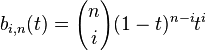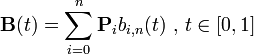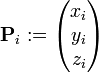# Welcome to the World of Bezier curves..

This blog is about the implementation of an editable Bezier Curve and the procedure to create a surface of revolution using the Bezier Curve.

The topic would sound confusing or tricky at first. Let me break it down in simpler steps of implementation.

I implemented the De Castuljau's algorithm for the Bezier curve implementation:

In the mathematical field of numerical analysis, De Casteljau's algorithm is a recursive method to evaluate polynomials in Bernstein form or Bézier curves, named after its inventor Paul de Casteljau. De Casteljau's algorithm can also be used to split a single Bézier curve into two Bézier curves at an arbitrary parameter value. The equations involved are as follows:

A Bézier curve B (of degree n, with control points ) can be written in Bernstein form as follows:where b is a Bernstein basis polynomialThe curve at point t0 can be evaluated with the recurrence relationThen, the evaluation of B at point t0 can be evaluated in n steps of the algorithm. The result B(t0) is given by :Moreover, the Bézier curve B can be split at point t0 into two curves with respective control points :When evaluating a Bézier curve of degree n in 3-dimensional space with n+1 control points Piand##### The Program:

The program contains a myinit(), display(), mymouse(), drawcurve(), fact() functions.

The main() function:

The main function takes the control points as inputs and stores them in an array. Using these control points we draw the Bézier curve.

• glutInit(&argc, argv);Initializes the GLUT.

• glutInitDisplayMode(GLUT_SINGLE | GLUT_RGBA);GLUT_SINGLE Bit mask to select a single buffered window. GLUT_RGBA selects the RGBA color model

• glutInitWindowSize(640, 480);glutCreateWindow(3D classroom);Creates a window with a title, initial width and height positioned at initial top-left corner.

• glutDisplayFunc(display);Registers display() as the re-paint event handler. That is, the graphics sub-system calls back display() when the window first appears and whenever there is a re-paint request.

• glutMouseFunc(myMouse);glutMouseFunc sets the mouse callback for the current window. When a user presses and releases mouse buttons in the window, each press and each release generates a mouse callback. The button parameter is one of GLUT_LEFT_BUTTON, GLUT_MIDDLE_BUTTON, or GLUT_RIGHT_BUTTON. For systems with only two mouse buttons, it may not be possible to generate GLUT_MIDDLE_BUTTON callback. For systems with a single mouse button, it may be possible to generate only a GLUT_LEFT_BUTTON callback. The state parameter is either GLUT_UP or GLUT_DOWN indicating whether the callback was due to a release or press respectively. The x and y callback parameters indicate the window relative coordinates when the mouse button state changed. If a GLUT_DOWN callback for a specific button is triggered, the program can assume a GLUT_UP callback for the same button will be generated (assuming the window still has a mouse callback registered) when the mouse button is released even if the mouse has moved outside the window.

• myinit();Invokes the myinit() once to perform all one-time initialization tasks.

• glutMainLoop();Finally, enters the event-processing loop.

One-Time Initialization Operations - myinit()

The myinit() function performs the one-time initialization tasks. It is invoked from main() once (and only once).

glClearColor(0.0f, 0.0f, 0.0f, 1.0f); // Set background color to black and opaque

glEnable(GL_COLOR_MATERIAL); // If enabled, have one or more material parameters track the current color

glEnable(GL_NORMALIZE); //If enabled and no vertex shader is active, normal vectors are normalized to unit length after transformation and before lighting.

glEnable(GL_DEPTH_TEST); // Enable depth testing for z-culling

glDepthFunc(GL_LESS); // Set the type of depth-test

We need to enable depth-test to remove the hidden surface, and set the function used for the depth test.

Calculating the points on curve - drawcurve(float t, struct point *p)

This function takes the control points as input and calculates the points on the bezier curve using the equations mentioned above. The value of t varies from 0.0 to 1.00 with interval width of 0.1

Drawing the curve - display()The red dots in the above picture show the control points and the grey curve is the Bézier curve through those points.

Drawing the control points in red:

glPointSize(5); // To set the pixels of the vertices

glBegin(GL_POINTS);// To start drawing points taken in main function

glEnd(); // End loop

glBegin(GL_LINE_STRIP);// To start drawing lines between points calculated in drawcurve()

glEnd(); // End loop

glTranslatef(1.5f, 0.0f, -7.0f); // Move right and into the screen

Dragging the control points - mymouse()

if(button==GLUT_LEFT_BUTTON && state==GLUT_DOWN) This is the condition using which we can start dragging a point when left mouse button is clicked we check in the stored control points if the clicked point is one among them. We keep the mouse button pressed and drag it to desired location where the condition becomes mouse button up, if(button==GLUT_LEFT_BUTTON && state==GLUT_UP) where the new control point is and we store it at the same index of the original control point.

glutPostRedisplay(); // marks the current window as needing to be redisplayed (refresh).

We store 11 points on the curve calculated in drawcurve() for task 4 to create surface of revolution.

The input is taken from the file test.txt generated in task 1,2 and stored in the plot structure, then the rot points are generated by using rot[i].x=plot[i].x * cos(j*(360/d)*(PI)/180); rot[i].y=plot[i].y; rot[i].z=plot[i].x * sin(j*(360/d)*(PI)/180);The angle of rotation d is taken from the user. There will be a total of d*10 vertices and d*9 faces and d*19 edges. The faces, edges and vertices are generated and appended in the sample.off file.